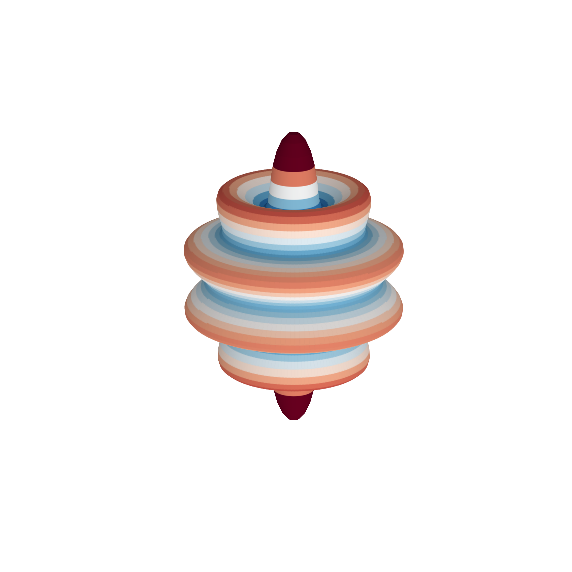# 3D Spherical Harmonic Plots¶

This notebook demonstrates how to generate a simple 3-dimensional plot of the data in an SHGrid class instance.

In :
%matplotlib inline

from pyshtools import SHCoeffs

lmax = 70
coeffs = SHCoeffs.from_zeros(lmax)
coeffs.set_coeffs(values=, ls=, ms=)

grid = coeffs.expand('GLQ')
grid.plot_3dsphere(elevation=20, azimuth=30);